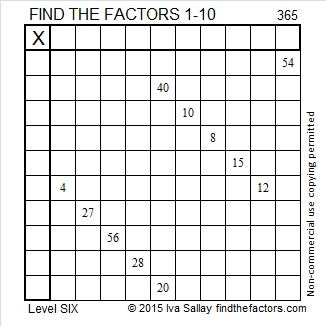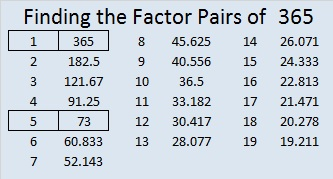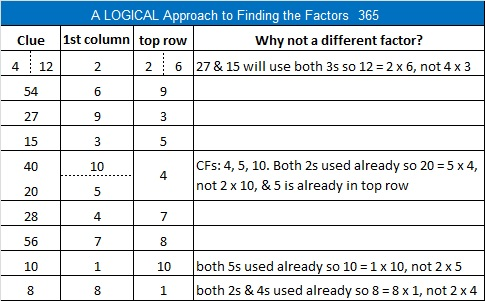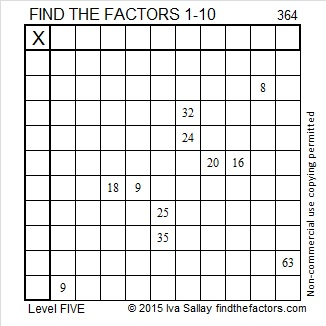# 365 and Level 6

If the earth revolved around the sun just a little bit faster there would be 364 days in a year, and those 364 days could easily be divided into 52-seven day weeks, thirteen 28-day months, and four 91-day seasons. If you were born on a Saturday, your birthday would occur on a Saturday every single year, too. Would there be more superstitions about the number 13 or less?

But that isn’t the way things are. It takes the earth nearly 365 1/4 days to revolve around the sun. We have settled on 365 days in a year with a 366-day leap year almost every four years.

365 has only two prime factors: 5 and 73. Seventy-three is a much bigger prime number than people use regularly, but it is only one more than 72 which has lots of great factors including twelve, a number that can easily be divided in half or into four seasons.

Besides if we could make the earth revolve around the sun faster, why not choose 360 days instead? Then we could have twelve 30-day months, four 90-day seasons, and our choice of 60 six-day weeks or 45 eight-day weeks. Perhaps we could listen to the Beatles sing Eight Days a Week.

On the other hand, I suppose we would all grow a little older a little faster…oops! Just forget I brought up the subject, please! And do something to keep your brain young, like this puzzle:Print the puzzles or type the factors on this excel file: 10 Factors 2015-01-19

• 365 is a composite number.
• Prime factorization: 365 = 5 x 73
• The exponents in the prime factorization are 1 and 1. Adding one to each and multiplying we get (1 + 1)(1 + 1) = 2 x 2 = 4. Therefore 365 has exactly 4 factors.
• Factors of 365: 1, 5, 73, 365
• Factor pairs: 365 = 1 x 365 or 5 x 73
• 365 has no square factors that allow its square root to be simplified. √365 ≈ 19.105# 364 and Level 5

364 is an easy tetrahedral number to remember because it is one less than the number of days in a year. It is the ridiculous sum total number of all the birds, rings, maids, dancers, and musicians given over the twelve days of Christmas.

One of 364’s factor pairs is also easy to remember: 7 × 52 = 364. There are 7 days in a week and 52 weeks in a year . . . or rather in a year minus one day.

364 is in this cool pattern:The factoring information for 364 is below the puzzle.Print the puzzles or type the factors on this excel file: 10 Factors 2015-01-19

• 364 is a composite number.
• Prime factorization: 364 = 2 x 2 x 7 x 13, which can be written 364 = (2^2) x 7 x 13
• The exponents in the prime factorization are 2, 1, and 1. Adding one to each and multiplying we get (2 + 1)(1 + 1)(1 + 1) = 3 x 2 x 2 = 12. Therefore 364 has exactly 12 factors.
• Factors of 364: 1, 2, 4, 7, 13, 14, 26, 28, 52, 91, 182, 364
• Factor pairs: 364 = 1 x 364, 2 x 182, 4 x 91, 7 x 52, 13 x 28, or 14 x 26
• Taking the factor pair with the largest square number factor, we get √364 = (√4)(√91) = 2√91 ≈ 19.079